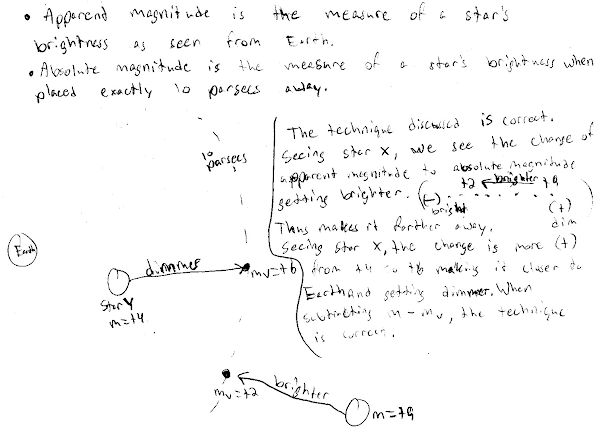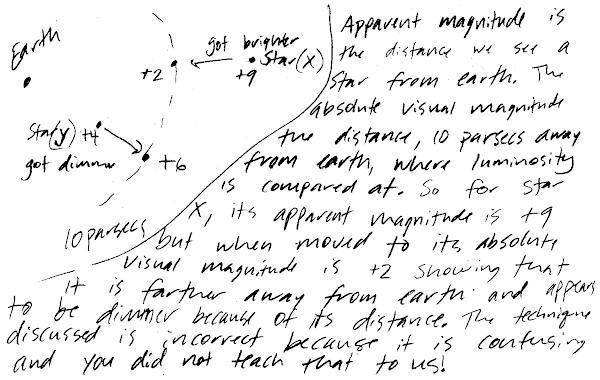## 20141213

### Astronomy midterm question: distance modulus comparison

Astronomy 210 Midterm 2, fall semester 2014
Cuesta College, San Luis Obispo, CA

An astronomy question on an online discussion board[*] was asked:
??: Two stars have these apparent magnitudes and absolute magnitudes. What can we say about their relative distances from Earth?
 mapparentmagnitude Mabsolutemagnitude Star X +9 +2 Star Y +4 +6

ba: The relative distances of stars can be determined by subtracting the absolute magnitude from the apparent magnitude. The more positive the answer, the farther away the star. For your example, Star X has a magnitude difference of 9 – 2 = +7, while the difference for Star Y is 4 – 6 = –2.
Discuss whether the technique discussed in this answer is correct or incorrect, and how you know this. Explain using the relationships between apparent magnitude, absolute magnitude, and distance.

• p:
Correct. Understands difference between apparent magnitude m (brightness as seen from Earth, when placed at their actual distance from Earth) and absolute magnitude (M (brightness as seen from Earth, when placed 10 parsecs away), and discusses:
1. that for star X, the brightness it has at its location (m = +9) is dimmer than its brightness when placed 10 parsecs away (M = +2), so its distance is greater than 10 parsecs; while for star Y, the brightness it has at its location (m = +4) is brighter than its brightness when placed 10 parsecs away (M = +6), so its distance is closer than 10 parsecs;
2. how this is consistent with the method proposed by "ba" (which follows directly from the "distance modulus" (mM) in the relation (mM) = 5·log(d) – 5), as star X has a distance modulus of +7 ("more positive the answer, the farther away the star") and star Y has a distance modulus of –2 (which would indicate that it is closer than star X).
• r:
Nearly correct (explanation weak, unclear or only nearly complete); includes extraneous/tangential information; or has minor errors. One of the two points (1)-(2) correct, other is problematic/incomplete.
• t:
Contains right ideas, but discussion is unclear/incomplete or contains major errors. Only one of the two points (1)-(2) correct, other is missing, or both are problematic.
• v:
Limited relevant discussion of supporting evidence of at least some merit, but in an inconsistent or unclear manner. At least attempts to use relationships between apparent magnitudes, absolute magnitudes, and distances.
• x:
Implementation/application of ideas, but credit given for effort rather than merit. Discussion based on garbled definitions of, or not based on proper relationships between apparent magnitudes, absolute magnitudes, and distances.
• y:
Irrelevant discussion/effectively blank.
• z:
Blank.
Section 70158
Exam code: midterm02s0vA
p: 14 students
r: 9 students
t: 12 students
v: 6 students
x: 4 students
y: 0 students
z: 0 students

A sample "p" response (from student 0978):A sample "t" response (from student 4743):